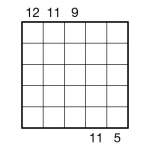Sum-Skyscraper 5×5 puzzle
I made this puzzle just before Christmas, and it’s been waiting on my desktop to be posted here ever since! Well, now it finally has been.This is a Sum Skyscraper. Place the digits 1 to 5 once each into every row and column in the grid. Numbers outside the grid provide the total (i.e. sum) of ‘visible’ grid digits along that row or column, if you imagine each digit as a building of that many storeys. Taller buildings always obscure shorter ones. So, for example, a clue for 21354 from the top of such a column would be 10, since the 2, 3 and 5 are visible (the 1 and 4 are obscured by the 2 and 5 respectively), and 2+3+5 = 10.